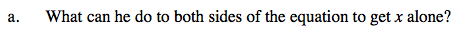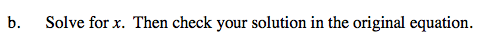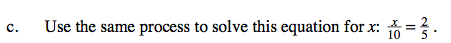Home > CAAC > Chapter 5 > Lesson 5.1.2 > Problem5-17

5-17.
1. When solving=for x, Nathan noticed that x is divided by 6. What can he do to both sides of the equation to get x alone? Homework Help ✎

1. Solve for x. Then check your solution in the original equation.

2. Use thesame process to solve this equation for x:=.Undo the division by multiplying by 6.$\frac{\textit{x}}{6}=\frac{5}{2}$

$\frac{x}{6}\cdot6=\frac{5}{2}\cdot6$

$\frac{6x}{6}=\frac{30}{2}$

x = 15

CHECK

$\frac{x}{6}=\frac{5}{2}$

If x = 15:

$\frac{15}{6}=\frac{5}{2}$

$15=\frac{5}{2}\cdot6$

$2\cdot15=30$

$30=30$Use the same methods shown in parts (a) and (b).